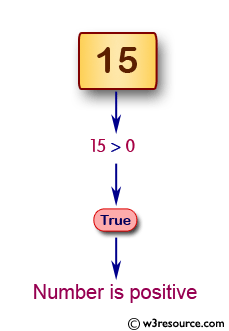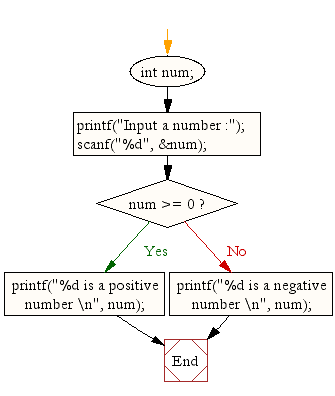﻿ C Program: Check whether a number is positive or negative - w3resource# C Exercises: Check whether a number is positive or negative

## C Conditional Statement: Exercise-3 with Solution

Write a C program to check whether a given number is positive or negative.

Pictorial Presentation:Sample Solution:

C Code:

``````#include <stdio.h>
void main()
{
int num;

printf("Input a number :");
scanf("%d", &num);
if (num >= 0)
printf("%d is a positive number \n", num);
else
printf("%d is a negative number \n", num);
}
```
```

Sample Output:

```Input a number :15
15 is a positive number
```

Flowchart:C Programming Code Editor:

Improve this sample solution and post your code through Disqus.

What is the difficulty level of this exercise?

Test your Programming skills with w3resource's quiz.

﻿

## C Programming: Tips of the Day

Why do C and C++ compilers allow array lengths in function signatures when they're never enforced?

It is a quirk of the syntax for passing arrays to functions.

Actually it is not possible to pass an array in C. If you write syntax that looks like it should pass the array, what actually happens is that a pointer to the first element of the array is passed instead.

Since the pointer does not include any length information, the contents of your [] in the function formal parameter list are actually ignored.

Ref : https://bit.ly/3fhlvdH